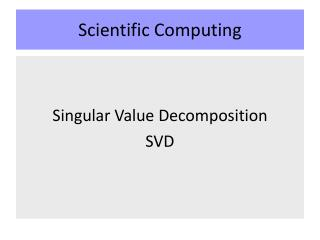DownloadDownload PresentationScientific Computing

# Scientific Computing

Download Presentation## Scientific Computing

- - - - - - - - - - - - - - - - - - - - - - - - - - - E N D - - - - - - - - - - - - - - - - - - - - - - - - - - -
##### Presentation Transcript

1. Scientific Computing Singular Value Decomposition SVD

2. SVD - Overview SVD is a way to decompose singular (or nearly singular) matrices, i.e. matrices that do not have inverses. This includes square matrices whose determinant is zero (or nearly zero) and all rectangular matrices.

3. SVD - Basics The SVD of a m-by-nmatrix A is given by the formula : Where : U is a m-by-m matrix of the orthonormal eigenvectors of AAT (U is orthogonal) VT is the transpose of a n-by-n matrix containing the orthonormal eigenvectors of ATA (V is orthogonal) D is a n-by-n Diagonal matrix of the singular valueswhich are the square roots of the eigenvalues of ATA

4. SVD • In Matlab: [U,D,V]=svd(A,0)

5. The Algorithm Derivation of the SVD can be broken down into two major steps  : • Reduce the initial matrix to bidiagonal form using Householder transformations (reflections) • Diagonalize the resulting matrix using orthogonal transformations (rotations) Initial Matrix Bidiagonal Form Diagonal Form

6. Householder Transformations Recall: A Householder matrix is a reflection defined as : H = I – 2wwT Where w is a unit vector with |w|2 = 1. We have the following properties : H = HT H-1 = HT H2 = I (Identity Matrix) If H is multiplied by another matrix, (on right/left) it results in a new matrix with zero’ed out elements in a selected row / column based on the values chosen for w.

7. Applying Householder To derive the bidiagonal matrix, we apply successive Householder matrices on the left (columns) and right (rows):

8. Application con’t From here we see : H1A = A1 A1K1 = A2 H2A2 = A3 …. AnKn = B [If m > n, then HmAm = B] This can be re-written in terms of A : A = H1TA1 = H1TA2K1T = H1TH2TA3K1T = … = H1T…HmTBKnT…K1T = H1…HmBKn…K1 = HBK

9. Householder Calculation Columns: Recall that we zero out the column below the (k,k) entry as follows (note that there are m rows): Let (column vector-size m) Note: Thus, where Ik is a kxk identity matrix.

10. Householder Calculation Rows: To zero out the row past the (k,k+1) entry: Let (row vector- size n) where wk is a row vector Note: Thus, where Ik is a (k-1)x(k-1) identity matrix.

11. Example To derive H1 for the given matrix A : We have : Thus, So,

12. Example con’t Then, For K1:

13. Example con’t Then, We can start to see the bidiagonal form.

14. Example con’t If we carry out this one more time we get:

15. The QR Algorithm As seen, the initial matrix is placed into bidiagonal form which results in the following decomposition : A = HBK with H = H1...Hnand K = Km…K1 The next step takes B and converts it to the final diagonal form using successive rotationtransformations (as reflections would disrupt upper triangular form).

16. Givens Rotations A Givens rotation is used to rotate a plane about two coordinates axes and can be used to zero elements similar to the householder reflection. It is represented by a matrix of the form : Note: The multiplication GA effects only the rows i and j in A. Likewise the multiplication AGt only effects the columns i and j.

17. Givens rotation The zeroing of an element is performed by computing the c and s in the following system. Where b is the element being zeroed and a is next to b in the preceding column / row. This results in :

18. Givens Example In our previous example, we had used Householder transformations to get a bidiagonal matrix: We can use rotation matrices to zero out the off-diagonal terms Matlab: [U,D,V]=svd(A,0)

19. SVD Applications Calculation of inverse of A: So, for mxn A define (pseudo) inverse to be: V D-1 Ut  : Given  : Multiply by A-1  : Multiply by V * : Multiply by D-1  : Multiply by UT  : Rearranging

20. SVD Applications con’t Condition number • SVD can tell How close a square matrix A is to be singular. • The ratio of the largest singular value to the smallest singular value can tell us how close a matrix is to be singular: • A is singular if c is infinite. • A is ill-conditioned if c is too large (machine dependent).

21. SVD Applications con’t Data Fitting Problem

22. SVD Applications con’t Image processing [U,W,V]=svd(A) NewImg=U(:,1)*W(1,1)*V(:,1)’

23. SVD Applications con’t Digital Signal Processing (DSP) • SVD is used as a method for noise reduction. • Let a matrix A represent the noisy signal: • compute the SVD, • and then discard small singular values of A. • It can be shown that the small singular values mainly represent the noise, and thus the rank-k matrix Akrepresents a filtered signal with less noise.